The Universe: Revolution

Space, as I have so far described it in these essays, provides 3 mutually perpendicular linear dimensions or degrees of freedom. However, it also facilitates revolution, or spin, about any three mutually perpendicular axes. This opens up a new mode of æthereal flow. [Portugês]

Revolution is the name of a kind of motion, which any object may have. It is the kind of motion in which the object moves, in a circle, around a centre. To be ped­antic, its path may not be exactly circular. It could, in general, be an ellipse or even a rosette shaped path, which is made up of successive open ellipses. Notwithstand­ing, revolu­tion is essentially an object travelling repeatedly around some point or centre. For example, the Earth revolves around the Sun in an annual cycle. The Moon revolves around the Earth in a monthly cycle.

Rotation, on the other hand, is when an object spins around an axis through the centre of the object itself. For example, the Earth rotates once a day. The Sun rotates ostensibly once every 26 days. However, any object is made up of smaller objects such as atoms. Thus, the atoms, of which the object is composed, are revolving around an axis through the centre of the object. Consequently, rotation is strictly a special case of revolution. It is merely collective revolution. Revolution is the fundament.

There are two ways in which an object can revolve. In other words, there are two modes of revolution. There is active revolution and there is passive revolution.

Passive revolution is where an object is revolving in a neutral state. This means that no real force is acting to sustain the object in its state of revolution. An exam­ple of passive revolution is the Earth revolving in orbit around the Sun.

Active revolution is where the exertion of a centripetal force is necessary in order to maintain the object in its state of revolution. A centripetal force is a real ext­ernally-applied force. It is not an inertial force. An example of active revolution is swinging a weight around your head on the end of a string. The string exerts a centripetal force upon the weight to keep it from flying off on a tangent.

Æthereal Flow

Before discussing the mechanisms of passive and active revolution, I wish to make a distinction in which a sink-hole is affected by æther flowing past it. Essentially, the universe comprises a flowing essence, which I have called æther. It flows con­tinually — at the velocity of light — from the outer reaches of the universe into minuscule entities, which I have called sink-holes. In a non-flowing state, æther does not exist. It is a flow or flux. This æthereal flux is what gives human con­sciousness the per­ception of time.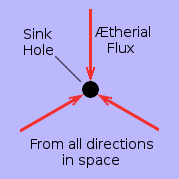Considered in isolation, a sink-hole is a conceptual hole in space into which the universal æther continually flows from all directions equally in 3-dimensional space. A sink-hole is not dynamically affected by its own incoming flow of æther. This is because, from its own point of view, its own in­coming flow is always symmetrical. It can only be per­turbed by an asymmetry in the flow of æther in its vicinity. In other words, it can only be physically acceler­ated by a net flow of æther which is flowing through it, across it or past it but not into it.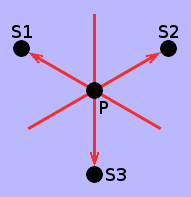However, if all the æther in the universe is flowing into sink holes, where does the æther, which flows through, across or past them, come from? The answer is, of course, the æther flowing into other sink holes. Across any point P in space, æther is flowing, in every direction, into what is ostensibly a homogeneous distribution of sink-holes S1, S2, S3 and so on, which populates the whole of space. Consequently, far from the immediate in­fluence of any local sink-hole, the flow of the æther acr­oss any point P in space is spherically symmetrical both to and from any direction.

This view of æthereal flow is what causes the inertial reaction to the forced acceler­ation of an object. In an orbital situation, it is what stops the planets or sink-holes falling towards each other along radial paths.

Thus, far from the immediate influence of any local sink-hole, the flow of æther into a sink hole and the flow of æther through, across or past a sink hole are both spherically symmetrical. Consequently, neither can have any perturbing effect on any sink hole. Notwithstanding, any asymmetry in the distribution of sink holes in a given locality causes a corresponding asymmetry in the æthereal flux passing thro­ugh or across any given point P in that locality. Any sink hole, at that point, will consequently suffer an acceleration in the direction of net æthereal flux.

Revolution, rotation and orbits involve two separate mechanisms which generate, respectively, convergent and divergent versions of æthereal flux asymmetry.

Free Mutual Attraction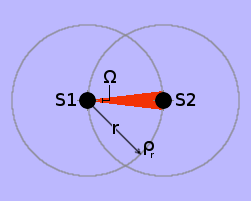The mechanism of mutual attraction between two sink holes, in an otherwise empty universe, is illus­trated on the right. However, only the mechanism whereby S1 attracts S2 is actually shown. Equally, S2 attracts S1, thus making the attraction mutual. The full explanation of this mechanism appears in my earlier essay: The Enigmatic Æther. Essential­ly, the spherically-sym­metrical in-flow of æther into S1 passes S2, creating an asymmetrical flux acr­oss S2, which causes S2 to accelerate towards S1.

Likewise, of course, the spherically-symmetrical in-flow of æther into S2 passes S1, creating an asymmetrical flux across S1, which causes S1 to accelerate towards S2. Note that in both cases, the æthereal flux flowing spherically-symmetrically into a sink hole, from all directions in space, plays no part in its own acceleration towards the other sink hole.

Please note my use of the word "accelerate" in the previous two paragraphs. It is important to note that this is passive acceleration. Although the sink holes app­roach each other with ever-increasing velocity through space, this is not as a result of a directed external force: the sink holes are merely "going with the flow" of the æther.

Any macroscopic object, such as a planet, is, according to my hypothesis, a vast homogeneous agglomeration of sink holes. So the mutual "gravitational" attraction between two planets is a result of the action of exactly the same mechanism as described above.

Restrained Mutual Attraction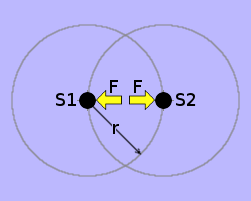Suppose it's possible to hold sink holes S1 and S2 in place so that they can't move. To do this, it is necessary to apply a real directed force F, to each sink hole, in the direction away from its attractor. Let's imagine that the forces are applied by the Fingers of God. The sink holes experience real dir­ected forces. But they are not accelerating thro­ugh space. Each is in exactly the same situ­ation as a person standing on the Earth exper­iencing the upward force of the ground on the bottoms of his feet.

In the absence of any external force, the two sink-holes each "go with the flow" of the other's in-flowing æther passing by. This causes the sink-holes to accelerate to­wards each other until they collide. However, if an external force F is applied to keep them apart, it causes an asymmetry in the flow of the æther past each of them.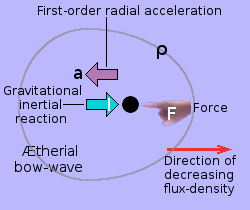Consider one of the sink-holes in isolation. The Finger of God exerts an external force F upon the sink-hole. This causes the sink hole to accelerate at a metres per second per second in the direction of the force. The sink hole is thus no longer "going with the flow" of its surrounding æther. This causes the flux density of the æther, flowing in the vicinity of the sink hole, to become spherically asym­metrical. This is depicted by the grey egg-shaped contour of constant flux density ρ in the diagram.

One way of looking at this situation is to imagine that, when a force F impedes the sink hole's natural tendency to "go with the flow" of the æther, it causes an æth­ereal bow-wave to form on the opposite side of the sink hole to that on which the Finger of God applies the force. This æthereal bow-wave produces the inertial re­action I.

I will, for the time being, leave this force F, exerted upon each sink hole by the Fingers of God, as a conceptual place-holder to be replaced by something concrete later on. However, in order to be able to construct this concrete replacement for F, it is first necessary to investigate the mechanism of constrained revolution.

Constrained Revolution

My favourite example of constrained orbit is the wheel-shaped space station in the film "2001: A Space Odyssey". The station's accommodation is inside a hollow torus-shaped tube. This forms the rim of a big wheel, which rotates slowly. The rotation causes the station's inhabitants within the tube to experience their own weight, as they would on Earth, thus allowing them to walk, sit, eat, work and rest normally. In conventional terms, the force of this "artificial gravity" acts outwards from the centre of rotation in all directions. People walk on the inner wall of the torus with their feet furthest from the centre of the wheel and their heads towards its centre.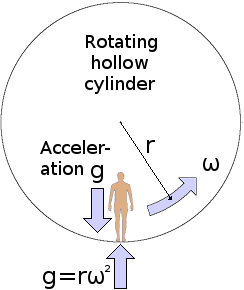The principle of constrained revolution, is shown on the left in terms of a hollow rotating cylinder in free space. To create the sensation of Earth's gravity, it is necessary to make rω² = g, in which r is the radius of the cylinder, ω is the angular velocity with which the cylinder is rotating and g is the so-called acceleration of gravity at the Earth's surface, which is 9·80665 metres per second per second. A cyl­inder with a radius of 100 metres would there­fore have to rotate at an angular velocity ω = √(g÷r) = √(9·80665÷100) = 0·313155712 radians per second to produce an "artificial gravity" of equal strength to that at the Earth's surface. This means that the cylinder would have to rotate once approximately every 20 seconds.

The so-called "gravitational acceleration", g, suffered by the body of a person standing on the wall of the rotating cylinder, is — according to the ideas I have been proposing throughout this series of essays — produced by the local radial flux-density gradient of the universal æther flowing radially outwards, at constant velocity c, through the person's body. To prevent the person's body from "going with the flow" of the æther, the inner surface of the wall of the cylinder must exert a centripetal force F = mrω², where m is the so-called "mass" of the person's body.

Of course, this so-called "artificial" gravity is not artificial: it is just as much a natural phenomenon as is the gravity of a planet. Notwithstanding, it is a very different natural phenomenon. The gravitational flux converges spherically into a planet from all directions in 3-dimensional space. The gravitational flux generated by rotation, on the other hand, diverges cylindrically. It diverges from a line: not from a point. That line is the axis of rotation through the centre of the cylinder. I think that this is a fundamentally important distinction.A large rotating cylinder with people walking, sit­ting, eating, working and resting inside its torus-shaped rim is systemically complicated. I must ab­stract the essence of this phenomenon of "rota­tional gravity" and encapsulate it in a simpler form. For this purpose, I shall replace the rotating cylin­der with a rotating dumbbell, as illustrated on the right, comprising two weights, joined together by a rigid weightless bar.

The weights are sufficiently small and far apart for any "gravitational" attraction be­tween them to be negligible compared with the centripetal force [pull] exerted upon them by the bar that joins them together.

Suppose that, while they are joined together by the rigid weightless rod, the two constrained weights are forcibly accelerated to an angular velocity ω. Once rotating at ω each weight suffers a real physical centripetal force, F = m† × r × ω², which is delivered through the rod and acts towards the centre of rotation.

† The constant m is known conventionally as the mass of the weight. However, as I have been proposing in this series of essays, m = k × n, where n is the number of æthereal sink-holes within the planet and k is a universal constant of proportion­ality.

Revolving Sink-Holes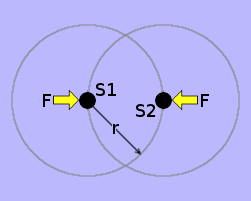The weights mentioned above are merely vast ag­glomerations of sink holes. I may therefore repl­ace each weight by a single sink hole. In doing so, however, I must also replace the centripetal force, exerted by the weightless bar, with a pair of in­ward opposing forces F exerted by the Fingers of God. The two sink holes are thus now in constrain­ed revolution around the common centre. In this case, each of the revolving weights comprises just one sink hole: i.e. n = 1.

The aspect of each sink-hole's revolutionary motion, which is the easiest for a hu­man being to perceive, is that the sink-hole is proceeding around a circular path at a constant velocity. However, æther is a velocity fluid. It does not manifest itself, in any way, to an object that is not accelerating. Consequently, this aspect of the sink-hole's motion cannot cause it to react in any way with the æther.

Notwithstanding, in a purely conceptual sense, the sink-hole is accelerating. Yet it is not acelerating through space — at least, not in the way in which nature has equip­ped human beings to perceive acceleration through space. This is because the sink-hole is accelerating in a direction which is perpendicular to its orbital track. The dir­ection in which the sink-hole is accelerating, as a result of its orbital motion, is rad­ially inwards towards its orbital centre.

The centripetal force Fp which is necessary to accelerate each sink hole sufficiently to constrain it to revolve around the common centre of revolution is given by:

 Fp = −½ × k × (n = 1) × r × ω² = −½krω²

The ½ is because r is twice the distance from a sink hole to the sink holes' common centre of revolution. The minus sign is because I have adopted the arbitrary con­vention that radially outward accelerations are positive and radially inward acceler­ations are negative.

How does the constraining of the two sink holes, by the forces F, to revolve about their common centre of revolution, affect the symmetry of the æthereal flux den­sity in the vicinity of each one?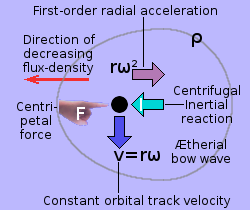Whenever external forces F are applied to two sink-holes, to keep them revolving around each other, the otherwise spherically symmetrical æth­ereal flux passing by each sink-hole becomes asymmetrical. This creates an up-stream density bow wave as depicted by the egg-shaped grey contour in the diagram on the right. However, each force F and its inertial reaction I now act in the opposite sense from what they did in the case of mutual attraction. The direction of decreasing æthereal density is also reversed, as is that of the æthereal bow-wave.

Note that, in the absence of any external forces F, the two "orbiting" sink-holes each "go with the flow". However, because they are not now accelerating in any way at all, each continues, at whatever relative velocity it has to its orbital partner, off at a straight tangent. The two sink-holes thus recede from each other forever.So far, I have ignored the real and present mutual attraction (discussed earlier) which the asymmet­rical flow of each sink hole's æther across the other sink hole exerts upon that other sink hole. This is recapped in the illustration on the left. As I explained in the Enigmatic Æther, the magnitude of this force Fa = Γ ÷ r², where Γ is a natural uni­versal constant. Compare this illustration with the previous one (above on right). The forces F, in each case, act in opposite directions.

They act against each other. The net radial force acting within a system comprising a pair of revolving mutually attracting sink holes is therefore given by:

 f = Fa + Fp = Γ ÷ r² − ½ × r × ω²

If f > 0 the sink holes fall towards each other in a decaying orbit.
If f = 0 the sink holes revolve about their common centre in a stable orbit.
If f < 0 the sink holes spiral away from each other in a expanding orbit.

For free orbit, the two place-holder forces F, represented by the Finger of God in the diagrams, are equal and opposite. Each is, in fact, the inertial reaction I (green arrow) in the opposing diagram. Thus, the two opposing inertial reactions are what holds the two sink-holes in mutual orbit around each other. This can only be pro­perly represented by combining the two diagrams and summing the two contours of constant æthereal flux density to create a single envelope.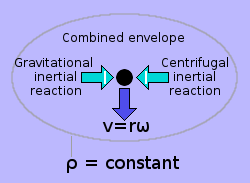The whole situation is clarified by the combined diagram on the right. The sink hole is shown in the dead centre of an ellipsoidal envelope of constant æthereal flux density ρ. This means that the cent­rifugal inertial reaction plus the so-called gravita­tional inertial reaction add up to zero. The æther­eal flux, passing by the sink-hole, is thus rarer al­ong the major axis of the ellipsoid — in both direc­tions — than it is in any other direction relative to the sink hole.

Each successive contour of constant density thus has the form of a prolate ellip­soid, with the sink-hole at the centre. This ellipsoid is, however, conically distorted, with the point of the distorting cone being at the sink-hole's centre of revolution.Successive contours of constant æthereal flux density, which surround a sink hole alone in free space, are spherical, as shown on the left. On the other hand, the successive con­tours of constant æthereal flux density, which surround a sink hole in orbit, are not spherical.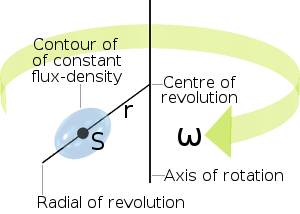Successive contours of constant æthereal flux density, which surround a sink hole in free orbit with another, around their com­mon centre of revolution, have the form of prolate ellipsoids. The major axes of these ellipsoids lie along the radial of revolution of the sink hole which they surround. Each sink hole lies at the centre of its concentric con­tours of constant flux density. The illustra­tion on the right shows the situation for just one of the orbiting sink holes.

So there's a difference between a sink hole in free space and a sink hole in free orbit. The latter's (would-be spherical) contours of equal flux density are squashed around the radial of revolution to form prolate ellipsoids. Furthermore, these ellips­oids are conically distorted by containing cones, whose points (or apexes) lie at the centre of revolution. Consequently, being in free orbit is not quite the same as being completely alone in free space.

Planets in Free OrbitConsider two identical planets freely orbiting each other around a common centre, as shown in the animation on the left. According to the æthereal hypothesis, which I have been building throughout this series of essays, these two planets are in blissful equilibrium, But they are not in equilibrium between two equal opposing real directed forces.

Instead, my hypothesis asserts that they are each in equilibrium between two opp­osing inertial reactions. And these two opposing inertial reactions result from two opposing æthereal flux density gradients.

Any macroscopic object, such as a planet, is, according to my hypothesis, a vast homogeneous agglomeration of sink holes. So the æthereal flux density contours surrounding a planet should be of the same form as those of a single sink hole. Consequently, for a solitary planet, far from the sight or influence of any other obj­ect, the successive contours of constant flux density are spherical. And for a planet in stable orbit, they have the form of prolate ellipsoids.

Convergent Acceleration

My term "convergent acceleration" refers to what most people at the present time think of as the spherically-convergent acceleration caused by gravitational attr­ac­tion.

According to the ideas I have been putting forward in these essays, a solid object comprises a vast number of æthereal sink-holes. If the object is rotating, all these sink-holes are orbiting the object's axis of rotation at the same angular velocity, ω. However, each sink-hole is doing so at its own particular radial distance from the object's axis of rotation, rn where the subscript n identifies one particular sink-hole within the object. The nth sink-hole is thus accelerating through the æther at rnω² metres per second per second.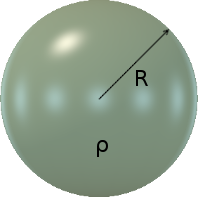Consider an object like the green sphere on the right. It is perfectly at rest in free space. In other words, it is not accelerating. It is not in orbit around any other object. It is not rotating. It has a radius of R metres. According to my postulation, the substance of the sphere, at its most fundamental level, is composed of sink-holes. I shall take the liberty of assuming that these are homogeneously distributed, at least down to a small molecular level of granularity. I shall therefore consider the sphere to have a homogeneous density of ρ sink-holes per cubic metre.

According to my hypothesis, a sink-hole, on the surface of the sphere will suffer an inertial acceleration a. This will be exactly countered by a real force, exerted by the rest of the sink-holes of the sphere, to stop the sink-hole in question from falling through "the ground" towards the centre of the sphere. Just like a person standing on the surface of the Earth suffers a downward acceleration of g. The magnitude of this inertial acceleration a on a single sink-hole located at the surface of the sphere is thus given by:
 But: a = G × M ÷ R² M = k × n n is the number of sink-holes in the object k is a universal constant of proportionality a = G × k × n ÷ R²       (1)

The number of sink-holes n, which makes up the substance of the sphere, is the volume V of the sphere times the sink-hole density ρ pertaining to the material of which the sphere is made.

Thus:   but: n = V × ρ V = (4/3) × π × R³ n = (4/3) × π × R³ × ρ       (2)

Substituting the expression for n given by equation (2) in equation (1):

 a = G × k × (4/3) × π × R³ × ρ ÷ R² = G × k × (4/3) × π × R × ρ

The quantities G, k and π are universal constants. I shall therefore make the ar­bitrary choice to represent the product of these constants, together with the con­stant 4÷3, by the Arabic letter خ as follows:

Let:   Thus: خ = G × k × (4/3) × π = خ × R × ρ       (3)

Divergent Acceleration

My term "divergent acceleration" refers to what most people at the present time think of as the cylindrically-divergent acceleration caused by centrifugal repulsion away from a centre of revolution.Suppose now that the green sphere is rotating, as shown on the left, around an axis passing through its centre (black line), at an angular velocity of ω radians per second. I have postulated that all mat­erial objects are made up of æthereal sink-holes. Almost all the sink-holes, of which the green sphere is composed, will effectively be revolving around the sphere's axis of rotation at angular velocity ω. However, they will, for the most part, be revolving at different distances from the sphere's axis of rotation. Any sink-hole within the sphere, which is at a given orbital distance r from the axis of rotation, will suffer an inertial acceler­ation of rω², which acts radially outwards from the axis of rotation.Consider the special case illustrated on the right of a sink-hole S, on the surface of the sphere, at the edge of the widest possible infinitely thin disc of radius R, whose plane is perpendicular to the sph­ere's axis of rotation. The sink-hole's spherically convergent inertial acceleration a = خRρ acts in­wardly towards the centre of the sphere, while the sink-hole's cylindrically divergent inertial acceler­ation, Rω², caused by the sphere's rotation, acts outwardly away from the sphere's axis of rotation. Consequently, there must exist an angular velocity ωf at which Rωf² becomes equal and opposite to خRρ, leaving the sink-hole itself in the weightless state of free orbit.

 Hence:   gives: a = Rخρ = −Rωf² dividing both sides by R ωf² + خρ = 0 Zero net inertial acceleration (4)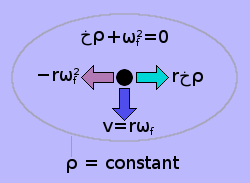The resulting deformation in shape of the sink hole's otherwise spherical contours of constant æthereal flux density is shown on the left. They have become ellipsoidal. The sink hole is at the centre of the ellipsoids. This means that the centri­fugal acceleration rωf² plus the so-called gravit­a­tional acceleration rخρ add up to zero. The æthe­real flux passing the sink hole is thus denser along the major axis of the ellipsoids than it is in any other direction.

 Note: if rωf² + rخρ > 0, the sink hole is off-set to the left within the ellipsoid. if rωf² + rخρ < 0, the sink hole is off-set to the right.

Of immediate interest here is that the angular velocity ωf, at which a sink-hole on the surface of the thin disc is in free orbit — i.e. weightless — is independent of the radius R of the sphere. The value of ωf is the same for any size of sphere made of the same material — or, more precisely, having the same sink-hole density, ρ.Now consider a sink-hole S, as shown on the right, within that same thin disc but not on its outer sur­face. This sink-hole is located at a radial distance r (less than R) from the sphere's axis of rotation. The spherically-symmetrical inward inertial accel­eration a, acting upon this sink-hole, is خ × ρ × r. This is exactly the same as for a smaller sphere of total radius r. This is because, within the sub-sphere (stronger blue) of radius r, the influence of all the sink-holes that lie outside it — i.e. between the radius r and the radius R (lighter blue) — can­cels to zero.

Hence:   giving: a = rخρ = −rωf² ωf² + خρ = 0   as before.

The upshot is that, when any sphere is rotating at angular velocity ωf, all the sink-holes within the largest infinitely thin disc passing through the sphere's centre and whose plane is perpendicular to the sphere's axis of rotation, become weightless. As I shall demonstrate later, they also lose inertia. Being without both weight and in­ertia implies that these sink-holes have become massless. This strongly suggests that the disc itself has become massless, or, at least, that mass itself isn't quite what we have always thought it to be.What about the sink-holes of the sphere that are outside this central thin disc? Consider a sink-hole S located on the sphere's surface at the edge of a smaller thin disc which is off-set along the axis of rotation at "latitude" θ from the sphere's "equ­ator". This situation is shown on the left. CL is a circle of longitude, which passes twice through the axis of rotation — once at each "pole". The smaller thin disc (green) has a radius of Rcosθ and is off-set from the centre of the sphere along its axis of rotation by a distance of Rsinθ. As always, the sink-hole S suffers a spherically-symmetrical in­ward inertial acceleration of خρ, along the radial R, towards the centre of the sphere.

However, the sink-hole's cylindrically-divergent outward inertial acceleration a is now ω² × R × sin(θ). But it does not now act in exact opposition to خρ. It acts out of line. To produce a sufficient outward acceleration to counter the inward accelera­tion خρ, the critical angular velocity ωf must be increased such that:

ωf² × R × cos(θ) = خ × ρ × R = خ × ρ ÷ cos(θ)

When θ is zero, cos(θ) is 1, so the formula becomes ωf² = خ × ρ, so the thin disc is not off-set at all. This situation is the special case discussed above. As θ increases, cos(θ) decreases, so ωf² must be greater. At the extreme, when the sink-hole S is at a pole — i.e. it is on the axis of rotation — cos(θ) becomes zero, making ωf² — and hence ω itself — infinite. Consequently, it can only ever be within part of a rotating sphere that "centrifugal" flux can overcome completely "gravitational" flux.Thus, any spinning object has both an inward æth­ereal flux asymmetry (bow wave) and an outward æthereal flux asymmetry (bow wave). These are depicted, as best as I am able to represent them, in the illustration on the right. The spinning object is spherical. This causes the profile of its æthereal flux asymmetry to have the form of an ellipsoidal disc. I have taken artistic license by showing the radius of the disc-shaped outward contour of constant flux density as being greater than that of the spherical inward contour of constant flux den­sity. However, this is purely to help to make them separately visible.

It should be well noted that the outward flux asymmetry is independent of the inward flux asymmetry. If I were to increase my object's rate of spin, this would not affect its incoming flux asymmetry.

The Fly-Wheel Principle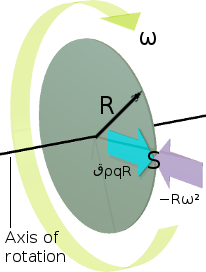The special case, described above, of the central thin disc of a rotating sphere, is of particular interest. This is because, within this thin disc, it is possible for the two opposing æthereal density gradients to be equal and therefore cancel each other out. I would therefore like to explore this special case further. To do this, I shall re­move the whole of the material of the sphere, except for this central thin spinning disc. The result is, in prin­ciple, the fly-wheel, as illustrated on the left, of radius R, rotating with angular velocity of ω radians per sec­ond around an axis which passes through its centre perpen­dicularly to the plane of the disc. The letter S signifies an arbitrary sink hole, located on the surface of the fly-wheel and which is itself part of the material of the fly-wheel.

The cyan (light blue) arrow in the diagram represents the radially outward accel­er­ation a suffered by the sink hole S as a result of the a real force exerted upon it by the material of the disc to oppose its natural tendency to "go with the flow" of the net æthereal flux flowing into all the other sink holes in the material of the disc.

Acceleration, = G × M ÷ R²  [an experimental observation] = k × n = G × k × n ÷ R² = V × ρ           [volume × sink hole density] = G × k × V × ρ ÷ R²

So far, this is the same as previously derived for the rotating sphere. However, the volume of a thin disc is not (4/3)πR³ but πR² × l, where l is the thickness of the fly-wheel disc.

So:   V = π × R² × l = l ÷ R  [the ratio between disc's thickness and radius] = π × R² × q × R = π × q × R³

The volume V of the disc is thus still, like as with the sphere, a function of the cube of the radius. So, substituting this expression for V in the previous expression for the acceleration:

Acceleration,   a = G × k × π × q × R³ × ρ ÷ R² = G × k × π × q × R × ρ = G × k × π   [a product of natural universal constants] = ق × ρ × q × R = قρqR

The ratio q here stands for the disc where the ratio (4÷3) stood for the sphere. However, because the thickness of a disc is arbitrary, I decided not to include it in the universal constant ق.

Note that the magnitude of the inward acceleration is proportional to the number of sink holes within the agglomeration of sink holes that forms the disc and its dir­ection is towards the centre of that agglomeration, namely, the centre of the disc. Thus, for any sink hole within the disc, the inward acceleration is the same as if all the substance of the disc were a sphere whose centre were coincident with the cen­tre of the disc. The magnitude of the acceleration, in the case of the disc, is there­fore given by the same expression قρqR as for a sphere of the same radius R.

The mauve arrow represents the radially outwards inertial acceleration −Rω² suff­ered by the sink hole S as a result of the sink hole's revolutionary motion around the disc's axis of rotation.

It is self-evident, therefore, that there must exist a particular angular velocity ωf at which the sink hole's centrifugal acceleration −Rωf² becomes equal and opposite to its inward "gravitational" acceleration قρqR, effectively placing the sink hole S in the weightless state of free orbit around the disc's axis of rotation. Thus, for any sink hole on the periphery of the disc:

 is the condition for free orbit. Divide both sides of this equation by R showing that the condition is independent of R.

This signifies that rotating the disc at the same angular velocity ωf will effectively put any sink hole within the material of the disc into the weightless state of free or­bit around the disc's axis of rotation. This merely means that the inward and out­ward æthereal flux-density gradients are the same for the fly-wheel as a whole.

What is the value of ωf in radians per second for a practical fly-wheel disc? Con­sider a disc weighing 20kg of radius ½ a metre. The acceleration "due to gravity", as it is conventionally called, at the surface of the disc is given by:

Acceleration,  a = G × M ÷ R²   ["gravitational"] = 6·67408 × 10-11 × 20 ÷ (0·5)² (1) = 5·339264 × 10-9 metres per second per second

How fast will the fly-wheel disc have to rotate to cancel out the effect of its own "gravity"? The "centrifugal" acceleration, as it is conventionally called, at the sur­face of the disc is given by:

Acceleration,  a = −R × ω²   ["centrifugal"] (2) = |a ÷ R|

For a sink hole on the outer surface of the disc at its maximum radius of 0·5 metre to be in "free orbit", the two accelerations must have the same magnitude. To express this condition, I can substitute the "gravitational" value for a given by expression (1) in expression (2).

 = 5·339264 × 10-9 ÷ 0·5 = 10·678528 × 10-9 = √(10·678528 × 10-9) = 0·000103337 radians per second = 0·000016447 hertz = 0·000986795 RPM

This means that the fly-wheel disc would have to rotate at the rate of once every 16 hours 53 minutes 23 seconds for its centrifugal effect to cancel out its gravitational attraction. Consequently, for all practical fly-wheel experiments, the value of قρqR is so extremely weak as to be well and truly negligible.

In contrast, within the scale of practical human experience, the term R × ω² has a very strong effect. In the space wheel mentioned earlier, to create the equivalent acceleration of the Earth's gravity, a 200 metre diameter space wheel need only rotate at a rate of once every 20 seconds. The rate of spin necessary for the 1 metre diameter fly-wheel disc, considered above, to accomplish a centrifugal ac­celeration equal to the acceleration of gravity on the Earth's surface is calculated below:acceleration, = −R × ω²   ["centrifugal"] = |−(g ÷ R)| = √(g ÷ R) = √(9·80665 ÷ 0·5) = 4·428690551 radians/second = 4·428690551 ÷ (2 × π) = 0·704847993 hertz = 42·290879561 RPM = 1/f = 1·418745616 seconds

The moving arrow, in the adjacent animation, shows the actual rate of spin (just over 42 RPM) necessary for the disc to generate a centrifugal acceleration equal to g, the acceleration due to the Earth's gravity at sea level. And as you can see, it is not very fast at all.

While rotating at this speed (just over 42 RPM), the disc's outward "centrifugal" acceleration is 1·83670446×109 (almost two billion) times its inward "gravitational" acceleration. Thus, on the practical human scale of fly-wheels, the "centrifugal" eff­ect is overwhelmingly dominant compared with its opposing "gravitational" effect. The two opposing accelerations can therefore only interact on a humanly perceiv­able scale for very massive objects (like stars or planets) which are rotating very slowly.

[An interesting point of practical reference is that the popular 720 mm diameter bicycle wheel has to rotate at only 49·8 RPM to generate the acceleration of gravity g at the outer surface of its tyre. That is equi­valent to riding the bike at just under 6·76 km/h — a slow jogging speed.]

Let us now return to consider the situation, within the disc itself, where the two opp­posing accelerations are not necessarily equal. That is, where ω ≠ ωf, which is where R(خρ + ω²) ≠ 0. Clearly, it is not now possible to divide both sides of this inequality by R. Consequently any non-zero value of R(خρ + ω²), for any given sink hole within the fly-wheel, depends on the radius R of the fly-wheel. In general, for a fly-wheel of radius R, there are 3 possible situations for a sink hole located at distance r from the fly-wheel's axis of rotation, where 0 ≤ r ≤ R, which are:

1)  r(خρ + ω²) > 0   Net real force acting radially outwards
2)  r(خρ + ω²) = 0   No net real force acting on the sink hole
3)  r(خρ + ω²) < 0   Net real force acting radially inwards

In case 1) above, the net force is equivalent to the force that acts upwards against the bottoms of your feet when you are standing on the Earth. Case 2) above is when the sink hole concerned is effectively in free orbit around the fly-wheel's axis of rotation. Case 3) above is a net force exerted by the material of the fly-wheel to hold the sink hole in place to stop it flying off at a tangent out of the fly-wheel.

The Gyroscopic Phenomenon

The one-metre diameter fly-wheel disc rotating, even at only 42 RPM, as described and illustrated in the animation above, exhibits certain kinds of behaviour that are quite counter-intuitive to common human experience.

Consider the fly-wheel disc as being in free space far away from the influence of any other object. It is not spinning. To it, the distant star field of the universe is not rotat­ing. The disc itself is not rotating. I have added to the disc a "massless" stub-shaft, which passes through the centre of the disc, perpendicular to its plane. The length of the stub-shaft is equal to the diameter of the disc. Thus the stub-shaft protrudes a distance R each side of the plane of the disc, where R is the radius of the disc, as shown in the following diagram.Let's apply a torque τ to the ends of the shaft as shown by the mauve coloured arrows. The torque τ is acting within a vertical plane which is per­pendicular to the plane of the disc. The torque is attempting to rotate the disc around its horiz­ontal diameter. This causes the disc to undergo an an­gular acceleration Ω' around its horizontal dia­meter. This angular acceleration will continue as long as the torque τ continues to be applied. If and when τ is removed, the disc will continue rotating at the angular velocity it attained during the time that the torque was applied. To slow the rotation of the disc back down to zero again, the same torque τ must be applied in the opposite sense for the same amount of time.

The fly-wheel disc thus exhibits, what is conventionally termed, angular momen­tum, just like a small electric grindstone, which takes time for the motor to rev it up to its operating speed and time for friction to slow it down until it stops after the motor is switched off. Of course, when accelerated in this bizarre way, around its horizontal diameter, the disc doesn't exhibit the same amount of rotational imped­ance as it would if accelerated around its shaft axis. This is because, in this case, more of the disc is rotating at a smaller distance from its axis of rotation. There's nothing coun­ter intuitive about this mode of rotation.Now set the disc rotating around its shaft axis at angular velocity ω, as shown on the right. The modest 4·4 radians per second, of the prev­ious animation, will suffice — just so long as this rate is maintained in the long term by some un­speci­fied means. Now apply the torque τ as be­fore. This time, the disc behaves differently. It does not rotate about the horizontal axis thro­ugh its diameter as before. Instead, it rotates about its vertical diametric axis, as indicated by the cir­cular blue arrow. Furthermore, it does not un­dergo an angular acceleration Ω'. Instead, it in­stantly acquires, and then continues to rotate at, a constant angular velocity Ω.

The angular velocity of Ω radians per second, taking place in the horizontal plane and indicated by the translucent blue arrow, is referred to as the fly-wheel disc's precession. The rate of precession (the value of Ω) is proportional to the value of the applied torque, τ. For example, if I double the torque, the rate of precession Ω doubles. The fly-wheel disc's rate of precession Ω is also inversely proportional to the angular velocity ω of the fly-wheel disc itself. For example, if I double the angular velocity ω of the fly-wheel disc, the rate of precession Ω halves.

The most significant observation for me, however, is that when the torque τ is removed, precession stops instantly. The fly-wheel disc does not keep on going as does a body in free space when a real directed force, which was accelerating it, is suddenly removed. Neither does the rate of precession Ω gradually slow down like the wheel of an electric grindstone does when it is switched off. Instead, precession stops instantly. The value of Ω instantly drops to zero.

To me, this implies that, in the horizontal plane at least, the fly-wheel disc has zero rotational inertia. Furthermore, since the torque τ cannot rotate the fly-wheel disc in the sense in which it (τ) is acting, the fly-wheel disc must have infinite rotational inertia around the torque axis. Of course, the fly-wheel disc still has rotational inertia around its axis of spin (its shaft axis). It would still require a considerable braking torque to kill the fly-wheel's spin.I find it very helpful to stare at the an­imation on the left while pondering on the above observations and their im­plications. Please click the animation for a larger view. One implication of the fly-wheel's apparent absence of rotational inertia around the vertical axis is as follows. Either, the disc has no angular inertia anyway within the horizontal plane of rotation, or, the disc's dynamic relationship with the universe does not change with the ap­plication or removal of the torque τ. In other words, the application of the tor­que τ does not accelerate the fly-wheel disc in any way.

The second option, according to my hypothesis, implies that the torque τ does not cause the fly-wheel to move relative to the æther. Whether the torque is being applied or not — whether the fly-wheel be precessing or not — does not change the fly-wheel's dynamic relationship with the rest of the universe. It is — and remains — in the same state of rest with respect to the rest of the universe.

The angular velocity ω of the fly-wheel disc and the angular velocity Ω of preces­sion are both rotational. Natural human intuition consequently led me (and others) to carelessly conclude that therefore the motive that produces the precession must also be rotational. That is, it must be a torque (or force-couple) τ and not merely a simple linear force F. But is this true to rational observation?

Torque or Force?

To answer this question, I must bring the precessing fly-wheel disc down to Earth, where it is subject to the Earth's gravity.Spin the fly-wheel up to an angular velo­city ω. Fix one free end of its shaft to a small cone-headed support stand via a universal ball or pin joint. The fly-wheel's rate of spin ω is maintained by unspeci­fied means. The fly-wheel is observed to precess around the support stand at ang­ular velocity Ω, as shown in the anima­tion on the right.

The fly-wheel appears to be suspended in thin air. Natural intuition would expect it to fall down. The end of the fly-wheel's half-shaft is simply resting on the point of the stand. It is not rigidly fixed to the stand. So it appears that the weight of the fly-wheel disc is being supported by some form of invisible cantilever fixed to the cone-headed stand. However, it is not even that simple because the measured up­ward force, exerted by the cone-headed stand, is only a small fraction of the fly-wheel's weight. In practical terms, it is probably no more than the weight of the shaft plus any non-rotating framework necessary for a practical apparatus. It ap­pears that the fly-wheel disc has "lost" its natural weight — the weight it has when simply resting on the ground.Conventional physics asserts that the fly-wheel is still being subjected to a torque τ. One half of τ's force-couple is thought to be the upward force, which the cone-headed support exerts upon the anchored end of the shaft. The other half-couple is imagined as an equal and opposite downward force ex­erted at the other end of the shaft, but which is, in really, thought to be double this force exerted downwards at the centre of the shaft by the weight of the fly-wheel.

Notwithstanding, the conventional theory is problematic. Nothing is exerting a real directed downward force upon the fly-wheel. A real directed downward force would have to be applied by something material at a specific point on the outer surface of the fly-wheel, which clearly isn't the case. Let us take this apparatus into free space, far away from the sight or influence of any other object. Here, the support stand is a rocket, which is applying a thrust equal to the force F exerted by the support stand on Earth. Observation suggests that the fly-wheel will precess in exactly the same way. The only force in the system is the linear real directed force exerted by the rocket. No torque is involved.

Although there is no real torque-partner to the force of the rocket's thrust, there is, nonetheless, an inertial reaction to it. Normally, the inertial reaction to a real linear directed force, accelerating an object through space, is directly in line with, and in the opposite sense to, that force. I see the spinning precessing fly-wheel disc as a special case of a simple real directed force accelerating an object through space.The thrust of the rocket exerts a force F, at the free end of the fly-wheel's shaft, per­pendicular to the fly-wheel's axis of rotation, as shown on the right. This causes the fly-wheel to precess around the force's line of action. An equal and opposite inertial re­action I to the force F must exist. It does. However, the rotating precessing fly-wheel appears to have off-set this inertial reaction laterally from the line of action of the force.

As can be seen from the diagram, the lateral off-set L of this equal and opposite inertial reaction I is the full length of the shaft. That is, it acts in a line that is as far again beyond the fly-wheel as the fly-wheel is from the point at which the rocket is applying the force F.

Let us now go back to the Earth-bound situation, with the fly-wheel precessing around the support stand. Another counter-intuitive observation is that, if you try to stop the fly-wheel in its orbit of precession, practically no force is required. It just stops instantly and drops down to the ground. This is true, even if the fly-wheel itself be quite "massive". The implication is that, while precessing, the fly-wheel has lost inertia. This deduction is substantiated by another observation. As the fly-wheel precesses around its orbit, there is practically no centripetal stretching force in the shaft. This implies that the motion of the fly-wheel around its orbit of precession produces little or no centrifugal "force". Thus, it would seem that the fly-wheel has lost its inertia — at least, both radially and tangentially to its orbit of precession.

I observed earlier that the spinning precessing fly-wheel had apparently lost its weight. It has also apparently lost its inertia along two mutually perpendicular axes which are both perpendicular to its would-be weight. Since, by convention, inertia and weight are both manifestations of the action of force upon mass, I think it is safe to conclude that the fly-wheel must have lost (at least most of) its mass.

The fly-wheel disc is thus being accelerated by the force F through space in line with the force. Neither the force F, nor its inertial reaction I, are able to rotate the fly-wheel about its diametric axis that is perpendicular to the line in which F acts. So F and I cannot form a torque. Consequently, the whole force + half-shaft + fly-wheel + half-shaft + inertial-reaction system behaves simply as a single object being accelerated linearly through space. However, and furthermore, while the fly-wheel is freely spinning and precessing, the effective "mass" of what is being linearly accelerated through space is only a vanishingly small fraction of the static "mass" of the fly-wheel.

All this is conventionally problematic. Notwithstanding, according to the theory I have been expounding in this series of essays, the fly-wheel did not have any mass in the first place, so it had none to lose. This is because what is conventionally per­ceived as mass is not a property of matter. It is a property of the relationship be­tween an object and the density-gradient field of the space-time (or æther) within the vicinity of the object.

I previously defined what is conventionally perceived as an object's mass m in terms of the number of sink holes n in the object times a universal constant which I called k. Notwithstanding, the spinning precessing fly-wheel, as described above, reveals that, although k may be universal, it is only constant for objects that are neither spinning nor precessing. If an object, such as the fly-wheel disc described above, is spinning and precessing, k varies with the fly-wheel's rate of spin ω, its rate of precession Ω, the radius r of the fly-wheel and the radius R of its orbit of pre­cession.

Thus: = kmax × function_of(ω, Ω, r, R)    [generally k << kmax†] = n × k = n × kmax × f(ω, Ω, r, R) = m × a    [one of Newton's observations] = F ÷ m    [where m is the apparent mass of the fly-wheel] = F ÷ {n × kmax × f(ω, Ω, r, R)}

† kmax is the value of k for a non-spinning non-precessing object.

This implies that, if the spinning fly-wheel were a large wheel-type space station, a small rocket mounted at the end of an axial tube, whose length was about equal to the space station's radius, could accelerate the space station as if it were a feather.

But this is all still counter-intuitive. It does not make sense in terms of general hu­man experience of motion. So what exactly is going on here? What supports the fly-wheel vertically? Why doesn't it just fall like a dead weight would? Is it possible to interpret this gyroscopic phenomenon in terms of intuitive concepts? I'll try.

Asymmetrical Angular VelocityThere is a significant asymmetry between the angular velocities of precession of the upper and lower halves of the fly-wheel. Consider a pair of diametrically opposite sink holes on the outer rim of the fly-wheel disc. Spend some time contemplating the motions of the two sink holes [small red and green spheres] in the adjacent animation. Com­pare their moti­ons as the fly-wheel spins and precesses.

In the above animation, the angular velocity of the fly-wheel's rotation ω is exactly the same as its angular velocity of precession Ω. When the fly-wheel's axis of rot­ation is in line with the observer, it can be clearly seen that the upper sink hole's angular velocity of precession is zero, while the lower sink hole's angular velocity of precession is very high. In fact, it is twice Ω. This occurs when the fly-wheel is closest to the observer (red sink hole at the top) and also when the fly-wheel is furthest from the observer (green sink hole at the top). In both cases where the fly-wheel is side-on to the observer, the two sink holes are at the same level. Con­sequently, they are both, at these points, precessing around the orbit at the same angular velocity Ω. At all intermediate positions around the orbit of preces­sion, the lower sink hole is always precessing faster, by some amount between zero and 2Ω.

The greatest difference between the angular velocities of precession of the upper and lower sink holes occurs at only two diametrically opposite points in the fly-wheel's orbit of precession. And it is the difference between the angular velocities, of the upper and lower sink holes, that is significant to the gyroscopic effect. Con­se­quently, the gyroscopic effect only occurs in full at these points. At the points in the orbit where the fly-wheel is side-on to the observer, there is no difference in the orbital speeds of the two sink holes and therefore no gyroscopic effect at all. Notwithstanding, since there is an astronomical number of sink holes spaced around the circumference of the fly-wheel, there are always sink holes at the very top and the very bottom of the fly-wheel. Consequently, the gyroscopic effect is apparent and smooth all the way around the fly-wheel's orbit of precession.Now consider the motion of just one sink hole in the rim of the fly-wheel, as illustr­ated in the animation on the right. The sink hole moves a considerable distance, around the orbit of precession, while it is in the lower half of its journey around the rim of the fly-wheel. How­ever, while in the upper half of its journey round the rim of the fly-wheel, the sink hole makes very little pro­gress around the orbit of precession.

In fact, in the animation, where ω=5×Ω, it makes a little progress in the backwards direction. Thus, we could use a common colloquial expression here to describe the progress of the sink hole. We can say that, during its every revolution around the rim of the fly-wheel, it's taking "3 steps forward and two steps backward" around the orbit of precession. This means that the sink hole's velocity, around the orbit of precession, is much greater when it is below the fly-wheel's shaft than when it is above the fly-wheel's shaft.

The total angular velocity Ωs of the sink hole around the orbit of precession thus varies cyclically with every complete turn of the fly-wheel. The cyclic motion of the fly-wheel's spin is thus modulating the cyclic motion of its precession around the support stand. The nature of this modulation is as follows.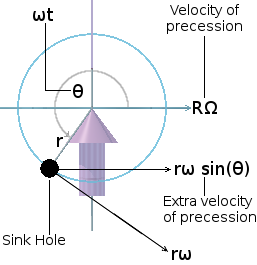In the adjacent diagram, the viewer is looking al­ong the fly-wheel's axis of rotation, from beyond the fly-wheel's orbit of precession, towards the support stand at the centre of the orbit. The fly-wheel is rotating anti-clockwise at an angular vel­ocity of ω radians per second. The fly-wheel's dir­ection of precession around its orbit is from left to right at a tangential velocity of RΩ metres per second, where R is the radius of the orbit in met­res. The sink hole's tangential velocity around the fly-wheel is rω metres per second, where r is the radius of the fly-wheel in metres. The com­ponent of the sink hole's tangential velocity rω in the direction of orbital precession is rω.sin(θ).

According to mathematical convention, the angle θ is measured from the positive horizontal direction (left to right). When 0 ≤ θ ≤ π, the sink hole is above the fly-wheel's axis of rotation. Over this range, sin(θ) is positive. When π ≤ θ ≤ 2π, the sink hole is below the fly-wheel's axis of rotation. Over this range, sin(θ) is nega­tive.

Thus the total tangential velocity: = R × Ω − r × ω × sin(θ) = Ω − (r ÷ R) × ω × sin(θ)

The minus sign is because the arithmetic value of r × ω × sin(θ) is negative when the velocities are in the same direction. This is entirely due to the convention according to which the angle θ is measured. Thus, for any sink hole on the rim of the fly-wheel, Ωs is greater when the sink hole is below the fly-wheel's axis of rotation and less when the sink hole is above the fly-wheel's axis of rotation.

In order for the sink hole to be constrained to follow the orbit of precession, a real directed force Fs towards the centre of the orbit must be continually applied to it. This real directed force is a stretching force along the fly-wheel's shaft. This force Fs is transmitted to the sink hole on the rim of the fly-wheel by the radial strip of material at the end of which the sink hole lies.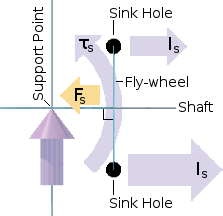The sink hole is law-bound to exert an equal and op­posite inertial reaction Is to the force Fs. As illustrated on the right, Is is much larger for a sink hole below the fly-wheel's axis of rotation than it is for a sink hole above it. The difference in Is for a sink hole below the fly-wheel's axis of rotation, and for a sink hole above it, forms an anti-clockwise torque around the fly-wheel's horizontal diameter, which the fly-wheel's shaft trans­lates into a torque τs around the support point. Now let us consider the cumulative effect of all the sink holes that make up the material of the fly-wheel.The inner sigma symbols, in the illustration on the left, are both the same. They sum the effects of all sink holes, within the material of the fly-wheel, which lie along an infinitely thin radial. That is, between q=0 and q=r, where q is a variable radius within the circle of the fly-wheel. The outer sigmas sum Is for all sink holes in the top half of the fly-wheel and all sink holes in the bottom half of the fly-wheel respectively.

The illustration is saying that, at any given time, the cumulative centrifugal inertial reaction of all the sink holes in the top half of the fly-wheel Itop is much less than the cumulative centrifugal inertial reaction of all the sink holes in the bottom half of the fly-wheel Ibot. That is, Itop << Ibot. This explains where the inertial torque comes from which enables the fly-wheel to maintain its shaft horizontal while it is rotating and precessing.

Although the above explains where the torque necessary to support the fly-wheel comes from, it gives no clue as to why this rotating precessing state of the fly-wheel should be stable. There is no doubt that this dynamic state is stable. After all, if I momentarily pulse the fly-wheel upwards or downwards, it nutates. It boun­ces back and then continues to bounce up and down, with ever-diminishing am­plitude, until its original state of smooth horizontal precession is re-established. This behaviour suggests to me that the whole dynamic system of the rotating pre­cessing fly-wheel must contain some form of self-stabilizing negative feed-back.

Before we consider the full ramifications of nutation, it will be useful to examine an­other aspect of the precessing fly-wheel phenomenon.

Tilted PrecessionSuppose a fly-wheel is precessing in the horizontal plane as shown on the right. I apply a constant force to the precessing end of its shaft. An equal reactive horiz­ontal force is resisted by the pivot. Thus, what is effectively be­ing applied to the ends of the fly-wheel's shaft is a torque in the sense shown by the blue arrow.

What does this applied torque cause to happen? It causes the shaft of the fly-wheel to tilt upwards, in a rotating vertical plane, with an angular velocity that is prop­ortional to the torque. Note that applying the torque [which is the rotation equi­valent of a force] does not cause the fly-wheel to undergo an upward angular accel­eration, as one might expect. As soon as the torque is relaxed, the fly-wheel tilts upwards no further.

A torque has moved through an angle. Consequently, energy has been expended. But the same energy needs to be expended again to bring the fly-wheel back to horizontal precession. It is thus the act of changing the tilt of the fly-wheel that absorbs the energy, not the new tilted state itself. The act of tilting must therefore be a transducer that conveys the energy somewhere else. But this is a digression. Notwithstanding how it is brought about, I will now simply consider this tilted state of precession in it own right.A spinning fly-wheel can adopt a mode of precession in which its shaft is tilted up­wards from the horizontal as shown on the left. Its orbit of precession, which is smaller than before, can be thought of as a circle of lati­tude ψ on an imaginary sphere whose equa­tor (the grey circle) was the fly-wheel's orbit of precession when its shaft was horiz­ontal. The plane of the orbit of the sink hole around the rim of the fly-wheel is now no longer parallel to the direction of the force exerted by the support stand.

As can be seen from the animation, the sink hole's radius of precession is now much greater during its lower half-cycle than during its upper half-cycle, thus increasing the ratio between Ibot and Itop. This seems to exactly compensate for the fact that the orbital radius is now only R.cos(ψ), instead of R as it was when the fly-wheel's shaft was horizontal.Now let us revert to considering the whole fly-wheel disc: not just a single sink hole on its outer rim. How can I cause a spinning fly-wheel to adopt this tilted mode of preces­sion? Starting with the fly-wheel disc prece­ssing with its shaft horizontal, how can I cause its shaft to lift so that it becomes tilted upwards at an angle ψ as shown on the right? I push the shaft round horizontally in the direction in which it is precessing. As I do so, the shaft tilts upwards by revolving around the support point in a direction per­pendicular to that in which I push it.

People mistakenly assume that what I am thereby doing is applying a torque in the horizontal plane: I supply one half of the torque's force-couple by pushing on the shaft, the other half-couple being the horizontal reaction of the support stand. But this isn't correct. I could achieve the same tilt by accelerating the support stand horizontally by applying a horizontal linear force to it, which turned in the horiz­ontal plane at the rate of precession. The linear inertial reaction to this force then causes the fly-wheel to precess additionally in a rotating vertical plane.

To achieve a permanent tilt angle of ψ to the horizontal, I apply this horizontal linear force to the support stand for only a small amount of time. Perhaps I could put the support stand on a small trolley with castor wheels, to which I attach a small rocket to supply a 200 millisecond burst of horizontal thrust exactly when the fly-wheel's horizontal shaft reaches the point in its precession at which it is per­pendicular to the direction of the rocket's thrust.The surprise is that the thrust the rocket needs to exert to elevate the fly-wheel is very small com­pared with the would-be dead weight W of the fly-wheel. The upward force Fψ exerted on the tilted shaft is greater than the upward force F0 was when the shaft were horizontal. Notwithstanding, it is still not equal to the dead weight W of the fly-wheel. I would guess that the ratio between W, Fψ and F0 somehow involves the cosine of the angle ψ. If I push the shaft in the direction of precession until it becomes vertical (ψ=½π), the upward force F½π on the support becomes the dead weight W of the fly-wheel.

It seems also that the fly-wheel's inertia is now more apparent. The inertial reaction Iψ is equal and opposite to the force Fψ, consequently, the inertia Iψ of the tilted fly-wheel is greater than the inertia I0 of the fly-wheel when its shaft is horizontal. It must then follow that the mass mψ of the fly-wheel when its shaft is tilted must be greater than the mass m0 of the fly-wheel when its shaft is horizontal. And in turn, the static mass m½π (what is conventionally perceived as the mass) of the fly-wheel must be greater than the mass mψ of the fly-wheel when its shaft is tilted.

Notwithstanding, to say that the mass of the fly-wheel varies does not give the complete picture. The mass of the fly-wheel, within the present context, is not a universal thing. It is the fly-wheel's apparent mass from the point of view of the applied force F. For this reason, perhaps it is better to imagine the rotating fly-wheel to be of fixed orientation and to imagine the support stand (or rocket) to tilted with respect to the fly-wheel's shaft. Then, from the point at the end of the shaft, the mass of the fly-wheel varies with the direction of the applied force.

The consequence of this observation is that mass cannot be a mere scalar quantity pertaining to the fly-wheel. It must be a vector field pertaining to the fly-wheel's relationship with the applied force. And the conduit of that relationship is the asym­metry in the æthereal flux, flowing into all the sink holes of the fly-wheel, caused by the application of the force.

In the previous two illustrations, I have shown the fly-wheel and its shaft enclosed inside a translucent sphere. This is firstly to show that the length of the fly-wheel's half-shaft is equal to that of its radius. Pretty well all gyroscope fly-wheel units are built to this proportion. It seems that when the half-shaft length is equal to the fly-wheel's radius, the gyroscopic effect is at its maximum, although I am not entirely sure about this. Secondly, the translucent sphere is a useful construct for consider­ing how the apparent mass of the fly-wheel varies with direction.

Envelope of PrecessionImagine the fly-wheel back in free space. The support stand is a rocket. If the fly-wheel is not rotating or precessing, the force F required to accelerate it at a fixed rate a is given by the equation F=m×a, where m is the mass of the fly-wheel. If it is rotating and precessing, the equation must be quali­fied with the sub­script ψ viz. Fψ=mψ×a be­cause the mass mψ of the fly-wheel decrea­ses as the ang­le ψ increases. Consequently, the force Fψ necessary to keep the fly-wheel ac­celerating at a constant rate of a must de­crease as the angle ψ increases.

I wager that for an ideal fly-wheel, its mass varies as the cosine of the angle ψ such that mψ=m0×cos(ψ), where m0 is the dead-mass of the fly-wheel. Here I define an ideal fly-wheel as having all its substance concentrated into an infinitely thin layer on its rim held by a weightless disc and half-shaft. The variable force Fψ required to keep the fly-wheel accelerating at a constant rate of a metres per second per sec­ond would then be given by Fψ=m0×cos(ψ)×a.

When the length L of the fly-wheel's half-shaft is greater or less than the radius R of the fly-wheel, the circular function cosψ would become an elliptical function ecosψ. In this sense, the circular function cosψ (where L = R) is really a special case of ecosψ (where L ≶ R).The size, shape and orientation of the en­velope, defining the limit of the fly-wheel's excursions within its orbit of precession, varies with the angle ψ at which the force Fψ is applied to one end of the fly-wheel's shaft. The way in which this envelope varies is illustrated in the animation on the left. The shape of the envelope varies from a horn torus (when ψ = 0) to a sphere (when ψ = ½π). The major and minor radii of the torus are both equal to the radius R of the sphere.

NOTE: A horn torus is a torus whose minor radius is equal to its major radius such that the would-be passage through its centre is only just closed, somewhat like the normal state of the anal sphincter.

When the angle ψ is zero, the half-shaft of the precessing fly-wheel sweeps out a disc, shown as a darker shade of translucent blue in the above animation. As ψ increases, the disc gradually closes into a cone of ever-decreasing angle until it closes completely onto the fly-wheel's shaft. At all values of ψ (between 0 and 90°) the cone represents the surface swept by the half-shaft of the precessing fly-wheel.

The lower conical arrow represents the real directed force Fψ exerted at the end of the fly-wheel's half-shaft. The upper conical arrow represents the equal and oppo­site inertial reaction Iψ to the force. The distance between the points of the two arrows is, for every value of ψ, proportional to the magnitude of the force Fψ requir­ed to keep the fly-wheel accelerating at a metres per second per second. This dist­ance is also the distance between the two cusps at which the diametrically opposite parts of the torus overlap and encroach into each other. This situation is where the major radius of the torus is actually less than its minor radius R.

NOTE: The foregoing assumes an ideal fly-wheel, as previously defined. Any real rotating object will invariably have a component of what I shall term dead-mass. This is essentially that part of the body which is either not rotating, or not rotating sufficiently, to be completely within the gyro­scopic state.

In the real world — or perhaps I should say: the real universe — rotating objects are never perfect fly-wheels. Most rotating objects, I would guess, are more of a spher­ical or oblate ellipsoidal shape. A real directed force can be applied only at one of the two points, on the surface of such an object, through which its axis of rotation passes. These points correspond to the ends of a fly-wheel's shaft. On the macro­scopic scale — the scale of stars and planets — the object itself can be seen and observed. On the microscopic scale — the scale of fundamental particles — the object cannot be seen or observed. If such a particle contained an internal rotating com­ponent, the most we could detect would be its acceleration, applied force and hence its apparent mass. We would be unaware of what was going on inside it.To any human observer, such a particle can only be the envelope of precession of whatever rotating element is inside it. Such a particle could possibly exhibit quite a wide range of what is conventionally perceived as mass. But whereas it exhibits its minimum "mass" along any convergent radial lying within a (2-dimensional) plane, it exhibits its maximum "mass" only directly along a single (1-dimensional) line. And it exhibits intermediate values of "mass" along an infinite series of conical surfaces.Consequently, the situations in which the particle ex­hibits its dead-weight mass to an applied force are very rare compared with those in which it exhibits very little mass. A particle can only be detected by somehow app­lying an external real directed force to it and observing its re­action to that applied force. Thus, a single entity of this kind will inevitably appear as any one of a cont­in­u­um of particles of different masses and shapes according to the relative direction from which a force is applied to it.

For any given direction ψ of an applied force, the envelope, of a rotating precessing object, could be thought of as the probability shell enclosing the space within which the rotating precessing object could be anywhere at any given instant. To me, this is loosely analogous to the notion of the probability shell of an electron within an atom. I think it would be interesting to investigate whether or not the envelope of a rotating precessing entity could possibly give rise to established observations of the nanoscopic world.

Mechanism of "Mass-Loss"

The means whereby the fly-wheel produces a torque, which acts in the sense nece­ssary to support it from one end of its shaft, has already been established. Notwith­standing, a very big problem remains. The amount of inertial reaction, generated by this torque, acting upon the support stand, is extremely small compared with that which would be necessary to cantilever the entire dead-weight of the fly-wheel. The small magnitude of this inertial reaction suggests that it is cantilevering the weight w of a much smaller mass m than the mass M required to account for the dead-weight W of the fly-wheel.

If one believes in mass as a property of matter then there are only two possibilities: either the fly-wheel's mass has become drastically reduced, or, it has, for the most part, become hidden from the observer's world. On the other hand, if one thinks of mass as a manifestation of the fly-wheel's relationship with the rest of the universe, there is another option for explaining the smallness of the inertial reaction which the end of the fly-wheel's shaft exerts upon the support stand. This is to consider how the profile of the æthereal flux density, for a sink hole on the rim of the fly-wheel, varies as it revolves around the fly-wheel's centre of rotation.The animation on the right is a repeat of the earlier animation showing two diametrically opposite sink holes on the rim of a fly-wheel whose angular velocity of rotation ω is the same as its angular velocity of precession Ω. In this animation, however, a contour of con­stant æthereal flux density (shown in trans­lucent blue) has been added for each of the two sink holes. Please notice how each sink hole's contour of constant flux density chan­ges shape as it revolves around the axis of the fly-wheel.

As a sink hole passes the uppermost point, its contour of constant flux density is spherical. As it passes the lowest point, its contour of constant flux density is at its most prolate. The orientation of the major axis of the prolate ellipsoidal contour of constant flux density lies along a line from the point of the support stand. The dir­ection of minimum flux density gradient lies along this line in the direction away from the support point.

As a sink hole descends from the uppermost point, its contour of constant flux density starts off spherical and becomes more and more prolate until it reaches the lowest point. As the sink hole subsequently ascends from the lowest point, its contour of constant flux density becomes less and less prolate until it once again becomes spherical at the top. But why does it do this?In the animation, I made Ω = ω. Consequently, each time a sink hole passes the uppermost point, it isn't actually revolving around any­thing. It is completely stationary with respect to the support point. This leaves the radial in-flow of its æthereal flux spherically sym­metrical. Thus, the æthereal flux density gradient, at any given radial distance from the sink hole, is the same in all directions.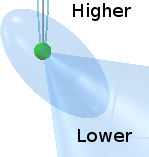Conversely, each time a sink hole passes the lowest point, it is moving at its maximum angular velocity 2Ω around the axis of the fly-wheel. As a result of the centrifugal inertial reaction thus generated, the radial in-flow of its æthereal flux is at its most asymmetrical. Thus, the æthereal flux density gradient, at any given radial distance from the sink hole, is different in different directions. Specifically, in all but a small solid angle of direc­tions, the æthereal flux density gradient is, in this case, very much higher.

More importantly, however, within the small solid angle centred on the sink hole and enclosing the outward radial, the æthereal flux density gradient is very much re­duced. This means that, from the point of view of an observer who is further than the sink hole from the centre of the fly-wheel, the apparent mass of the sink hole is very much reduced. In other words, it appears to be a lot less than unity.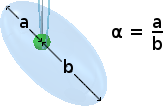Consequently, the inertial reaction I of the sink hole to any real directed force, applied in the upward direction, is sig­nificantly less. In effect, from the point of view of this for­ce, my universal constant, which I have previously called k, is effectively multiplied by the asymmetry ratio α of the out­ward to inward radial flux density gradients. The ratio α is of course fractional.

Consider the rotating precessing fly-wheel to be precessing around its support stand in Earth's gravity. Since ω=Ω, the combined precession rate of 2Ω resolves as a revolution of the sink hole around the point of the support stand. So, because the length of the fly-wheel's half-shaft is equal to the radius of the fly-wheel, the radius of revolution joining the sink hole and the point of the support stand is at 45° to the horizontal (or the vertical). Hence, the prolate ellipsoidal contours of constant flux density gradient is inclined at 45°, as shown in the above animation and the subse­quent diagrams.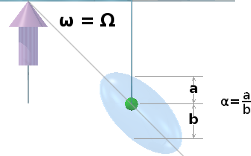The proportional weight loss of the fly-wheel is the same as its "mass loss" ratio α in the up­ward vert­ical direction. So the relevant value of α is the ratio a:b where a and b are measured along the vertical radial of the fly-wheel as shown on the right. Of course, this only applies when the sink hole is at the bottom of the fly-wheel. The value of α for all the sink holes at all other places within the material of the fly-wheel must be greater.

Consequently, the combined "mass-loss" of the fly-wheel as a whole can only be arrived at by a rather complicated process of integration, which must take into account the varying contributions of ω and Ω for all the different radii and angular positions at which each sink hole, within the material of the fly-wheel, lies.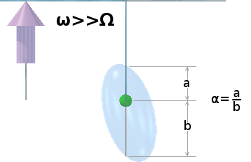As can be seen from the above diagram, when ω=Ω (when the prolate ellipsoidal contour of con­stant flux density lies at 45° to the vertical), the distances a and b are not very different. So α is a fairly large fraction. Consequently, the effective "mass-loss" of the fly-wheel is not very great. However, if we rev up the fly-wheel to make ω very much greater than Ω, the contour tilts much more towards the vertical, making α a much smal­ler fraction.

This has the effect of reducing the apparent mass of the fly-wheel — and hence its weight — to a much greater extent. This, in turn, correspondingly reduces the up­ward force, which the support point must exert upon the end of the half-shaft, in order to support the rotating precessing fly-wheel.

A Self-Regulating State

A fly-wheel, which is rotating and precessing at smooth constant angular velocities (ω and Ω), is in a stable dynamic state. This stable dynamic state is an attractor. This means that, should the fly-wheel be perturbed from this smooth constant dynamic state by some external influence, it will automatically gravitate back to its original smooth constant dynamic state. In so doing, the rotating precessing fly-wheel will dissipate, into its environment, any energy it received from whatever per­turbed it.

Perturbing the fly-wheel momentarily, in its direction of spin, causes its angular velocity of rotation ω to increase. However, this results in a corresponding reduc­tion in its angular velocity of precession Ω, such that its overall angular momentum of rotation plus precession does not change. So there is no change in its overall en­ergy. The perturbing pulse, in this case, is met by very little inertial reaction.

Perturbing the fly-wheel momentarily, in its direction of precession, causes the fly-wheel to move to an inclined orbit (ψ>0). This, in turn, produces a corresponding increase in the downward inertial reaction exerted by the end of the fly-wheel's half-shaft upon the support stand. Although this results in an increase in the fly-wheel's so-called potential energy (which is relative), it does not impart any absolute energy to the fly-wheel's rotating precessing state. The perturbing pulse, in this case, is also met by very little inertial reaction.

Notwithstanding, any attempt to momentarily perturb the fly-wheel in the direction perpendicular to both its spin and its precession is met with a very strong inertial reaction. This inertial reaction is truly a reactance and not a resistance. In other words, it does not, itself, directly dissipate the energy of the perturbing impulse. Rather, it stores the energy imparted by the impulse. And it stores this energy in the dynamic form of a mechanical oscillation. This form of mechanical oscillation is called nutation.The phenomenon of nutation is illustrated by the adjacent animation. A perturbing pul­se is momentarily applied downwards at the free end of the shaft. This produces an inst­ant­aneous increase of δΩ in the fly-wheel's ang­ular velocity of precession. So the fly-wheel is now precessing at an angular velo­city of Ω + δΩ. This new angular velocity of preces­sion is now too fast for the horizontal orbit to be stable.

NOTE: In this discussion, I assume that the fly-wheel precesses round a support stand in Earth's gravity. However, it could equally well be in free space, away from the influence of any other objects, with the support stand replaced by a rocket. The rocket maintains a thrust F to sustain the precession of the fly-wheel, which is countered by an equal and opposite inertial reaction I from the fly-wheel. The momentary pulse is provided by an extra short sharp burst of thrust δF from the rocket, which is counter­ed by an equal and opposite inertial pulse δI from the fly-wheel.

This increase δΩ in the fly-wheel's velocity of precession creates a corresponding increase δτs in the upward torque on the fly-wheel. However, the externally-applied downward pulse is short-lived. When it finishes, the fly-wheel's angular velocity of precession drops instantly back to Ω. Notwithstanding, the increase in torque δτs, which has now disappeared, has imparted an upward angular momentum to the fly-wheel. Consequently, the fly-wheel is now travelling upwards, away from its stable horizontal orbit of precession.

Having insufficient angular velocity of precession to maintain a higher orbit, the fly-wheel eventually loses all its upward momentum and falls back down again to its stable orbit. However, as it passes its stable orbit again, it has the reverse of its original upward momentum supplied by the short-lived additional upward torque δτs. Consequently, the fly-wheel overshoots its stable orbit in a similar way to that in which a pendulum overshoots its vertical rest position. The fly-wheel therefore continues downwards to a lower orbit for which its angular velocity of precession Ω is again inadequate. So the fly-wheel starts to "fall" upwards back towards its stable horizontal orbit. This process repeats, with the fly-wheel oscillating (nutating) up­wards and downwards, above and below its stable horizontal orbit, in what app­ears ostensibly to be a sinusoidal motion.

To illustrate this motion clearly, the above animation shows this oscillation as in­def­initely sustained. In reality, however, the oscillatory motion of nutation is heavily damped. It decays rapidly, with the fly-wheel quickly reverting to its original smooth stable state of continuous precession around its horizontal orbit. In this sense, it is less of the nature of a swinging pendulum and more like the audible ring of a bell or the damped oscillation of a resonant electrical circuit containing a large element of pure resistance. This rapid damping is, in effect, the necessary negative feed-back mechanism that pulls the perturbed fly-wheel back to its original stable dynamic state.

The energy, of the original perturbing pulse, is thus dissipated quite rapidly by the process of nutation. To my mind, this rate of damping cannot be accounted for by friction alone. In fact, I think that mechanical friction can account for only very little of the damping that occurs. Consequently, most of the energy, delivered by the original perturbing pulse, must be dissipated by some other mechanism, which is clearly not thermal nor any other kind of electromagnetic radiation.

This suggests to me that the impedance, which damps the nutational oscillation, must be more reactive than resistive. In other words, the process of nutation does not, itself, dissipate the energy but, instead, converts the energy into some other form. Since this energy is not readily perceivable by human sense or instrument, I am led to believe that it must be radiated as an æthereal flux-density wave. It effec­tively modulates the density-gradient of the flux of the passing æther which is flow­ing into every sink hole in the universe.

The momentary pulse causes sink holes in the fly-wheel to accelerate in directions perpendicular to the plane of the fly-wheel. This causes an elongation in the con­tours of constant flux-density surrounding each sink hole. This, in turn, causes a momentary shift in the magnitude and direction of the flux density gradient sur­rounding the sink hole, which gives rise to a radiating æthereal flux density pulse, which effectively radiates away from the sink hole.

A pulse of such modulation occurs whenever the fly-wheel is momentarily perturb­ed from any stable orbit. And this is true not only for the orbit in which the fly-wheel's shaft is horizontal (perpendicular to the force exerted by the support stand) but for all orbits at any inclination ψ to the horizontal.

Conclusion

In this essay, I have tried to show, however tenuously, that the basic concept of a universal æther, flowing into sink holes, can underpin the phenomena observed in revolutionary and rotational motion.

The conceptual model I have built, throughout this series of essays, is neither rig­or­ous nor complete. But it is — for the most part — internally consistent.

My conceptual model is in no way consistent with the mainstream views of reality. But then the Standard Model of Physics and the Theory of Relativity are not consist­ent with each other.

My objective, in this series of essays, has not been to construct a new all singing and dancing alternative theory of everything to compete with the mainstream. My inter­est is not primarily in Physics. It is in the nature of human perception. It is in the various constructs or frameworks, which the human mind uses, to try to under­stand the universe to which its physical senses provide it with a raw view.

Many people think that, what they see as the laws of physics as expressed in math­ematical formulas, are inherent to the universe. I do not agree. I think that all the laws, observed by man, are constructs that are entirely within the mind of man. They are simply frameworks, or systems of categorization, which the mind of man constructs around his observations, as a means of trying to get a handle on what he sees. The æthereal flow model, which I have constructed through this series of essays, is such a framework. And it works for me.

I have, on this web site, written essays about other personal views of the universe, which do not attempt to be consistent with the view expounded in this series of essays, or even with each other. But this mutual inconsistency is not a problem for me. This is because I know that none of my views are the universe. They are just means by which I can get some feel for the conscious experience I am still having of a vast environment in which I arrived almost 75 years ago. And that's OK.

It makes me realise that chasing an evermore elusive view, of ever-finer detail, of the physical universe is not what life is about. It is about constantly improving the way I, as a conscious entity, relate with other human beings.

© Aug 2016 to Jan 2017 Robert John Morton | PREV